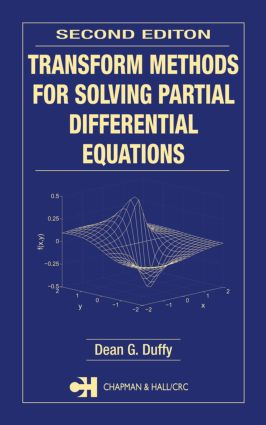# Transform Methods for Solving Partial Differential Equations

## 2nd Edition

Chapman and Hall/CRC

728 pages | 294 B/W Illus.

Hardback: 9781584884514
pub: 2004-07-15
SAVE ~\$43.99
\$219.95
\$175.96
x
eBook (VitalSource) : 9780429147050
pub: 2004-07-15
from \$110.00

FREE Standard Shipping!

### Description

Transform methods provide a bridge between the commonly used method of separation of variables and numerical techniques for solving linear partial differential equations. While in some ways similar to separation of variables, transform methods can be effective for a wider class of problems. Even when the inverse of the transform cannot be found analytically, numeric and asymptotic techniques now exist for their inversion, and because the problem retains some of its analytic aspect, one can gain greater physical insight than typically obtained from a purely numerical approach.

Transform Methods for Solving Partial Differential Equations, Second Edition illustrates the use of Laplace, Fourier, and Hankel transforms to solve partial differential equations encountered in science and engineering. The author has expanded the second edition to provide a broader perspective on the applicability and use of transform methods and incorporated a number of significant refinements:

New in the Second Edition:

· Expanded scope that includes numerical methods and asymptotic techniques for inverting particularly complicated transforms

· Discussions throughout the book that compare and contrast transform methods with separation of variables, asymptotic methods, and numerical techniques

· Many added examples and exercises taken from a wide variety of scientific and engineering sources

· Nearly 300 illustrations--many added to the problem sections to help readers visualize the physical problems

· A revised format that makes the book easier to use as a reference: problems are classified according to type of region, type of coordinate system, and type of partial differential equation

· Updated references, now arranged by subject instead of listed all together

As reflected by the book's organization, content, and many examples, the author's focus remains firmly on applications. While the subject matter is classical, this book gives it a fresh, modern treatment that is exceptionally practical, eminently readable, and especially valuable to anyone solving problems in engineering and the applied sciences.

### Reviews

“This book provides a detailed account of the application of transform methods to solving linear PDEs in science and engineering. The main techniques studied are the Fourier and Laplace transform, Hankel transforms, and the Wiener-Hopf technique. In this second edition the references have been extended and numerical and asymptotic techniques are included. It provides a valuable source of concrete examples from diverse fields of applications with detailed solutions.”

— M. Kunzinger

“The discussed solutions typically involve quite difficult algebra, while non-trivial mathematical steps, such as a change of order of integration or expansion into infinite series (product) are not justified. This gives the book more of an ‘engineering’ flavour; nonetheless it is certainly of interest to a wider interest.”

“The book contains many examples and exercises taken from a wide variety of scientific and engineering sources. Also numerical examples are presented using Matlab to help readers for better understanding of the physical problems.”

—Studia Univ. “Babes-Bolyai” Mathematica, Sept. 2005

THE FUNDAMENTALS

Fourier Transforms

Laplace Transforms

Linear Ordinary Differential Equations

Complex Variables

Multivalued Functions, Branch Points, Branch Cuts, and Riemann Surfaces

Some Examples of Integration which Involve Multivalued Functions

Bessel Functions

What are Transform Methods?

METHODS INVOLVING SINGLE-VALUED LAPLACE TRANSFORMS

Inversion of Laplace Transforms by Contour Integration

The Heat Equation

The Wave Equation

Laplace's and Poisson's Equations

Papers Using Laplace Transforms to Solve Partial Differential Equations

METHODS INVOLVING SINGLE-VALUED FOURIER AND HANKEL TRANSFORMS

Inversion of Fourier Transforms by Contour Integration

The Wave Equation

The Heat Equation

Laplace's Equation

The Solution of Partial Differential Equations by Hankel Transforms

Numerical Inversion of Hankel Transforms

Papers Using Fourier Transforms to Solve Partial Differential Equations

Papers Using Hankel Transforms to Solve Partial Differential Equations

METHODS INVOLVING MULTIVALUED LAPLACE TRANSFORMS

Inversion of Laplace Transforms by Contour Integration

Numerical Inversion of Laplace Transforms

The Wave Equation

The Heat Equation

Papers Using Laplace Transforms to Solve Partial Differential Equations

METHODS INVOLVING MULTIVALUED FOURIER TRANSFORMS

Inversion of Fourier Transforms by Contour Integration

Numerical Inversion of Fourier Transforms

The Solution of Partial Differential Equations by Fourier Transforms

Papers Using Fourier Transforms to Solve Partial Differential Equations

THE JOINT TRANSFORM METHOD

The Wave Equation

The Heat and Other Partial Differential Equations

Inversion of the Joint Transform by Cagniard's Method

The Modification of Cagniard's Method by De Hoop

Papers Using the Joint Transform Technique

Papers Using the Cagniard Technique

Papers Using the Cagniard-De Hoop Technique

THE WIENER-HOPF TECHNIQUE

The Wiener-Hopf Technique When the Factorization Contains No Branch Points

The Wiener-Hopf Technique when the Factorization Contains Branch Points

Papers Using the Wiener-Hopf Technique

WORKED SOLUTIONS TO SOME OF THE PROBLEMS

INDEX